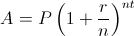×

# Compound Interest Calculator

 Principal: Annual interest rate: % Years: Frequency of compounding: Compound Continuously Compound Daily Compound Monthly Compound Quarterly Compound Semi-Annually Compound Annually

## About Compound Interest Calculator

The online Compound Interest Calculator is used to calculate the compound interest and see how your money can grow over time with compound interest.

## Compound Interest

Compound interest means that each time interest is paid, it is added to or compounded into the principal and thereafter also earns interest. This addition of interest to the principal is called compounding. Compound interest may be contrasted with simple interest, where interest is not added to the principal.

## Compound Interest Formulas

The following is the compound interest formula for periodic compounding:Where:
A = final amount
P = principal amount (initial investment)
r = annual nominal interest rate
t = number of years
n = number of compounding periods per year (for example, 12 for monthly compounding)

If the compounding is continuous, the calculation will be:Where:
A = final amount
P = principal amount (initial investment)
r = annual nominal interest rate
t = number of years
e = 2.7182818284

Our website uses cookies to improve your user experience. If you continue browsing, we assume that you consent to our use of cookies. More information can be found in our privacy policy
Deals